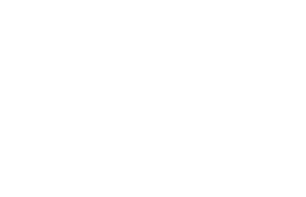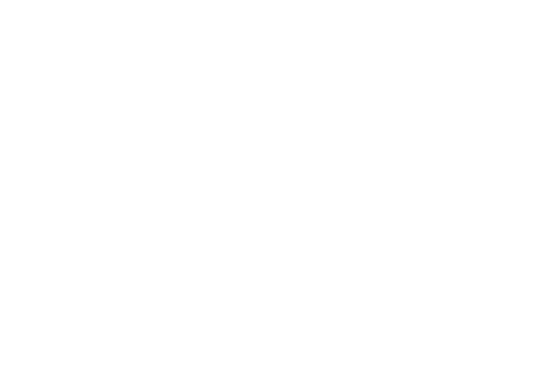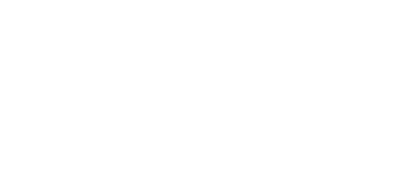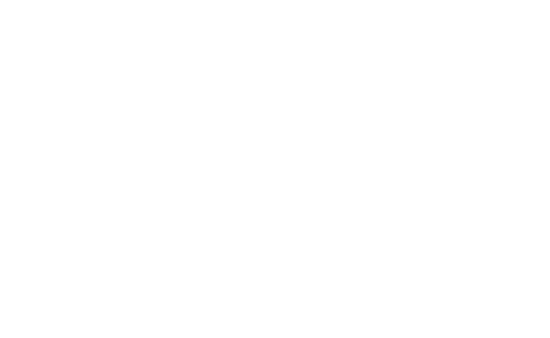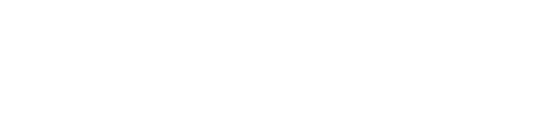DIY全攻略 (上) ：架子搭起来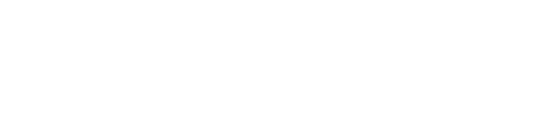https://raw.githubusercontent.com/auduno/clmtrackr/dev/build/clmtrackr.js

`1<!doctype html>`

`2<html>`

`3<body>`

`4 <script src="https://code.jquery.com/jquery-3.3.1.min.js"></script>`

`5 <script src="https://cdn.jsdelivr.net/npm/@tensorflow/[email protected]"></script>`

`6 <script src="clmtrackr.js"></script>`

`7 <script src="main.js"></script>`

`8</body>`

`9</html>`

`1<video id="webcam" width="400" height="300" autoplay></video>`

`1\$(document).ready(function() {`

`2 const video = \$('#webcam');`

`3`

`4 function onStreaming(stream) {`

`5 video.srcObject = stream;`

`6 }`

`7`

`8 navigator.mediaDevices.getUserMedia({ video: true }).then(onStreaming);`

`9});`

`1const ctrack = new clm.tracker();`

`2ctrack.init();`

`1ctrack.start(video);`

`1<canvas id="overlay" width="400" height="300"></canvas>`

`2<style>`

`3 #webcam, #overlay {`

`4 position: absolute;`

`5 top: 0;`

`6 left: 0;`

`7 }`

`8</style>`

`1const overlay = \$('#overlay');`

`2const overlayCC = overlay.getContext('2d');`

`3`

`4function trackingLoop() {`

`5 // Check if a face is detected, and if so, track it.`

`6 requestAnimationFrame(trackingLoop);`

`7`

`8 let currentPosition = ctrack.getCurrentPosition();`

`9 overlayCC.clearRect(0, 0, 400, 300);`

`10`

`11 if (currentPosition) {`

`12 ctrack.draw(overlay);`

`13 }`

`14}`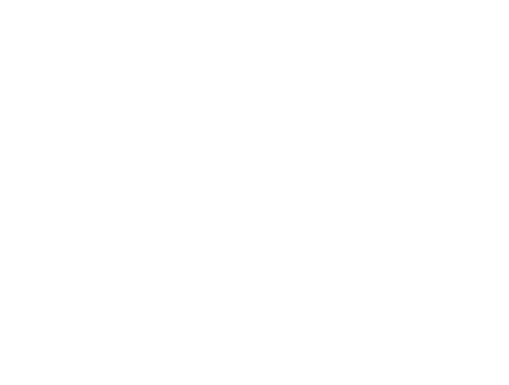cmltrackr很善良，除了画个轮廓之外，还有 70 个面部特征，我们可以选择自己需要的部分。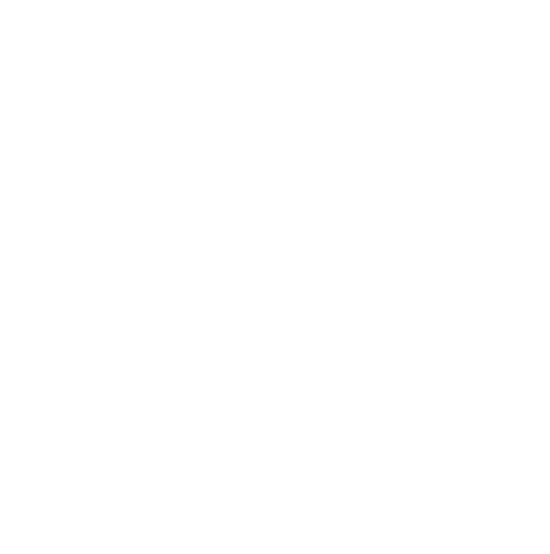`1<canvas id="eyes" width="50" height="25"></canvas>`

`2<style>`

`3 #eyes {`

`4 position: absolute;`

`5 top: 0;`

`6 right: 0;`

`7 }`

`8</style>`

`1function getEyesRectangle(positions) {`

`2 const minX = positions - 5;`

`3 const maxX = positions + 5;`

`4 const minY = positions - 5;`

`5 const maxY = positions + 5;`

`6`

`7 const width = maxX - minX;`

`8 const height = maxY - minY;`

`9`

`10 return [minX, minY, width, height];`

`11}`

`1if (currentPosition) {`

`2 // Draw facial mask on overlay canvas:`

`3 ctrack.draw(overlay);`

`4`

`5 // Get the eyes rectangle and draw it in red:`

`6 const eyesRect = getEyesRectangle(currentPosition);`

`7 overlayCC.strokeStyle = 'red';`

`8 overlayCC.strokeRect(eyesRect, eyesRect, eyesRect, eyesRect);`

`9`

`10 // The video might internally have a different size, so we need these`

`11 // factors to rescale the eyes rectangle before cropping:`

`12 const resizeFactorX = video.videoWidth / video.width;`

`13 const resizeFactorY = video.videoHeight / video.height;`

`14`

`15 // Crop the eyes from the video and paste them in the eyes canvas:`

`16 const eyesCanvas = \$('#eyes');`

`17 const eyesCC = eyesCanvas.getContext('2d');`

`18`

`19 eyesCC.drawImage(`

`20 video,`

`21 eyesRect * resizeFactorX, eyesRect * resizeFactorY,`

`22 eyesRect * resizeFactorX, eyesRect * resizeFactorY,`

`23 0, 0, eyesCanvas.width, eyesCanvas.height`

`24 );`

`25}`

DIY全攻略 (下) ：训练与测试

#### 1 追踪鼠标

`1// Track mouse movement:`

`2const mouse = {`

`3 x: 0,`

`4 y: 0,`

`5`

`6 handleMouseMove: function(event) {`

`7 // Get the mouse position and normalize it to [-1, 1]`

`8 mouse.x = (event.clientX / \$(window).width()) * 2 - 1;`

`9 mouse.y = (event.clientY / \$(window).height()) * 2 - 1;`

`10 },`

`11}`

`12`

`13document.onmousemove = mouse.handleMouseMove;`

#### 2 捕捉图像

TensorFlow.js提供了一个助手函数，叫 tf.fromPixels() ，只要用它来储存第二张画布里走出的图像，然后归一化：
`1function getImage() {`

`2 // Capture the current image in the eyes canvas as a tensor.`

`3 return tf.tidy(function() {`

`4 const image = tf.fromPixels(\$('#eyes'));`

`5 // Add a batch dimension:`

`6 const batchedImage = image.expandDims(0);`

`7 // Normalize and return it:`

`8 return batchedImage.toFloat().div(tf.scalar(127)).sub(tf.scalar(1));`

`9 });`

`10}`

`1const dataset = {`

`2 train: {`

`3 n: 0,`

`4 x: null,`

`5 y: null,`

`6 },`

`7 val: {`

`8 n: 0,`

`9 x: null,`

`10 y: null,`

`11 },`

`12}`

`13`

`14function captureExample() {`

`15 // Take the latest image from the eyes canvas and add it to our dataset.`

`16 tf.tidy(function() {`

`17 const image = getImage();`

`18 const mousePos = tf.tensor1d([mouse.x, mouse.y]).expandDims(0);`

`19`

`20 // Choose whether to add it to training (80%) or validation (20%) set:`

`21 const subset = dataset[Math.random() > 0.2 ? 'train' : 'val'];`

`22`

`23 if (subset.x == null) {`

`24 // Create new tensors`

`25 subset.x = tf.keep(image);`

`26 subset.y = tf.keep(mousePos);`

`27 } else {`

`28 // Concatenate it to existing tensors`

`29 const oldX = subset.x;`

`30 const oldY = subset.y;`

`31`

`32 subset.x = tf.keep(oldX.concat(image, 0));`

`33 subset.y = tf.keep(oldY.concat(mousePos, 0));`

`34 }`

`35`

`36 // Increase counter`

`37 subset.n += 1;`

`38 });`

`39}`

`1\$('body').keyup(function(event) {`

`2 // On space key:`

`3 if (event.keyCode == 32) {`

`4 captureExample();`

`5`

`6 event.preventDefault();`

`7 return false;`

`8 }`

`9});`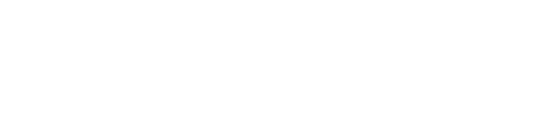TensorFlow.js里面有一个和Keras很相似的 API 可以用。

`1let currentModel;`

`2`

`3function createModel() {`

`4 const model = tf.sequential();`

`5`

`6 model.add(tf.layers.conv2d({`

`7 kernelSize: 5,`

`8 filters: 20,`

`9 strides: 1,`

`10 activation: 'relu',`

`11 inputShape: [\$('#eyes').height(), \$('#eyes').width(), 3],`

`12 }));`

`13`

`14 model.add(tf.layers.maxPooling2d({`

`15 poolSize: [2, 2],`

`16 strides: [2, 2],`

`17 }));`

`18`

`19 model.add(tf.layers.flatten());`

`20`

`21 model.add(tf.layers.dropout(0.2));`

`22`

`23 // Two output values x and y`

`24 model.add(tf.layers.dense({`

`25 units: 2,`

`26 activation: 'tanh',`

`27 }));`

`28`

`29 // Use ADAM optimizer with learning rate of 0.0005 and MSE loss`

`30 model.compile({`

`31 optimizer: tf.train.adam(0.0005),`

`32 loss: 'meanSquaredError',`

`33 });`

`34`

`35 return model;`

`36}`

`1function fitModel() {`

`2 let batchSize = Math.floor(dataset.train.n * 0.1);`

`3 if (batchSize < 4) {`

`4 batchSize = 4;`

`5 } else if (batchSize > 64) {`

`6 batchSize = 64;`

`7 }`

`8`

`9 if (currentModel == null) {`

`10 currentModel = createModel();`

`11 }`

`12`

`13 currentModel.fit(dataset.train.x, dataset.train.y, {`

`14 batchSize: batchSize,`

`15 epochs: 20,`

`16 shuffle: true,`

`17 validationData: [dataset.val.x, dataset.val.y],`

`18 });`

`19}`

`1<button id="train">Train!</button>`

`2<style>`

`3 #train {`

`4 position: absolute;`

`5 top: 50%;`

`6 left: 50%;`

`7 transform: translate(-50%, -50%);`

`8 font-size: 24pt;`

`9 }`

`10</style>`

`1<button id="train">Train!</button>`

`2<style>`

`3 #train {`

`4 position: absolute;`

`5 top: 50%;`

`6 left: 50%;`

`7 transform: translate(-50%, -50%);`

`8 font-size: 24pt;`

`9 }`

`10</style>`

`1<div id="target"></div>`

`2<style>`

`3 #target {`

`4 background-color: lightgreen;`

`5 position: absolute;`

`6 border-radius: 50%;`

`7 height: 40px;`

`8 width: 40px;`

`9 transition: all 0.1s ease;`

`10 box-shadow: 0 0 20px 10px white;`

`11 border: 4px solid rgba(0,0,0,0.5);`

`12 }`

`13</style>`

`1function moveTarget() {`

`2 if (currentModel == null) {`

`3 return;`

`4 }`

`5 tf.tidy(function() {`

`6 const image = getImage();`

`7 const prediction = currentModel.predict(image);`

`8`

`9 // Convert normalized position back to screen position:`

`10 const targetWidth = \$('#target').outerWidth();`

`11 const targetHeight = \$('#target').outerHeight();`

`12 const x = (prediction.get(0, 0) + 1) / 2 * (\$(window).width() - targetWidth);`

`13 const y = (prediction.get(0, 1) + 1) / 2 * (\$(window).height() - targetHeight);`

`14`

`15 // Move target there:`

`16 const \$target = \$('#target');`

`17 \$target.css('left', x + 'px');`

`18 \$target.css('top', y + 'px');`

`19 });`

`20}`

`21`

`22setInterval(moveTarget, 100);`